# Photogrammetric Solutions

## Producer Field Guide

HGD_Product
Producer Field Guide
HGD_Portfolio_Suite
Producer

As stated previously, digital photogrammetry is used for many applications, ranging from orthorectification, automated elevation extraction, stereopair creation, feature collection, highly accurate point determination, and control point extension.

For any of the aforementioned tasks to be undertaken, a relationship between the camera or sensor, the image or images in a project, and the ground must be defined. The following variables are used to define the relationship:

• Exterior orientation parameters for each image
• Interior orientation parameters for each image
• Accurate representation of the ground

Well-known obstacles in photogrammetry include defining the interior and exterior orientation parameters for each image in a project using a minimum number of ground control points (GCPs). Due to the costs and labor intensive procedures associated with collecting ground control, most photogrammetric applications do not have an abundant number of GCPs. Additionally, the exterior orientation parameters associated with an image are normally unknown.

Depending on the input data provided, photogrammetric techniques such as space resection, space forward intersection, and bundle block adjustment are used to define the variables required to perform orthorectification, automated DEM extraction, stereopair creation, highly accurate point determination, and control point extension.

Space Resection

Space resection is a technique that is commonly used to determine the exterior orientation parameters associated with one image or many images based on known GCPs. Space resection is based on the collinearity condition. Space resection using the collinearity condition specifies that, for any image, the exposure station, the ground point, and its corresponding image point must lie along a straight line.

If a minimum number of three GCPs is known in the X, Y, and Z direction, space resection techniques can be used to determine the six exterior orientation parameters associated with an image. Space resection assumes that camera information is available.

Space resection is commonly used to perform single frame orthorectification, where one image is processed at a time. If multiple images are being used, space resection techniques require that a minimum of three GCPs be located on each image being processed.

Using the collinearity condition, the positions of the exterior orientation parameters are computed. Light rays originating from at least three GCPs intersect through the image plane through the image positions of the GCPs and resect at the perspective center of the camera or sensor. Using least squares adjustment techniques, the most probable positions of exterior orientation can be computed. Space resection techniques can be applied to one image or multiple images.

Space Forward Intersection

Space forward intersection is a technique that is commonly used to determine the ground coordinates X, Y, and Z of points that appear in the overlapping areas of two or more images based on known interior orientation and known exterior orientation parameters. The collinearity condition is enforced, stating that the corresponding light rays from the two exposure stations pass through the corresponding image points on the two images and intersect at the same ground point. The figure below illustrates the concept associated with space forward intersection.

Space Forward Intersection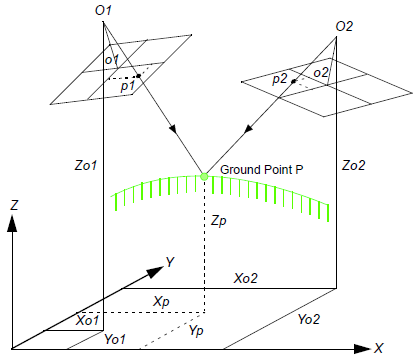Space forward intersection techniques assume that the exterior orientation parameters associated with the images are known. Using the collinearity equations, the exterior orientation parameters along with the image coordinate measurements of point p on Image 1 and Image 2 are input to compute the Xp, Yp, and Zp coordinates of ground point p.

Space forward intersection techniques can be used for applications associated with collecting GCPs, cadastral mapping using airborne surveying techniques, and highly accurate point determination.

For mapping projects having more than two images, the use of space intersection and space resection techniques is limited. This can be attributed to the lack of information required to perform these tasks. For example, it is fairly uncommon for the exterior orientation parameters to be highly accurate for each photograph or image in a project, since these values are generated photogrammetrically. Airborne GPS and INS techniques normally provide initial approximations to exterior orientation, but the final values for these parameters must be adjusted to attain higher accuracies.

Similarly, rarely are there enough accurate GCPs for a project of 30 or more images to perform space resection (that is, a minimum of 90 is required). In the case that there are enough GCPs, the time required to identify and measure all of the points would be costly.

The costs associated with block triangulation and orthorectification are largely dependent on the number of GCPs used. To minimize the costs of a mapping project, fewer GCPs are collected and used. To ensure that high accuracies are attained, an approach known as bundle block adjustment is used.

A bundle block adjustment is best defined by examining the individual words in the term. A bundled solution is computed including the exterior orientation parameters of each image in a block and the X, Y, and Z coordinates of tie points and adjusted GCPs. A block of images contained in a project is simultaneously processed in one solution. A statistical technique known as least squares adjustment is used to estimate the bundled solution for the entire block while also minimizing and distributing error.

Block triangulation is the process of defining the mathematical relationship between the images contained within a block, the camera or sensor model, and the ground. Once the relationship has been defined, accurate imagery and information concerning the Earth’s surface can be created.

When processing frame camera, digital camera, videography, and nonmetric camera imagery, block triangulation is commonly referred to as aerial triangulation (AT). When processing imagery collected with a pushbroom sensor, block triangulation is commonly referred to as triangulation.

There are several models for block triangulation. The common models used in photogrammetry are block triangulation with the strip method, the independent model method, and the bundle method. Among them, the bundle block adjustment is the most rigorous of the above methods, considering the minimization and distribution of errors. Bundle block adjustment uses the collinearity condition as the basis for formulating the relationship between image space and ground space. IMAGINE Photogrammetry Project Manager uses bundle block adjustment techniques.

In order to understand the concepts associated with bundle block adjustment, an example comprising two images with three GCPs with X, Y, and Z coordinates that are known is used. Additionally, six tie points are available. The figure below illustrates the photogrammetric configuration.

Photogrammetric Configuration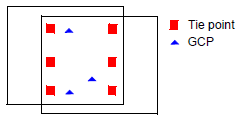Forming the Collinearity Equations

For each measured GCP, there are two corresponding image coordinates (x and y). Thus, two collinearity equations can be formulated to represent the relationship between the ground point and the corresponding image measurements. In the context of bundle block adjustment, these equations are known as observation equations.

If a GCP has been measured on the overlapping areas of two images, four equations can be written: two for image measurements on the left image comprising the pair and two for the image measurements made on the right image comprising the pair. Thus, GCP A measured on the overlap areas of image left and image right has four collinearity formulas.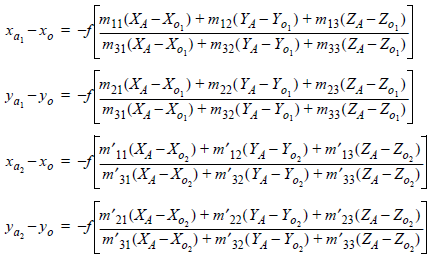One image measurement of GCP A on Image 1: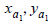One image measurement of GCP A on Image 2: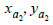Positional elements of exterior orientation on Image 1: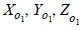Positional elements of exterior orientation on Image 2: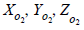If three GCPs have been measured on the overlap areas of two images, twelve equations can be formulated, which includes four equations for each GCP (see the "Photogrammetric Configuration" figure in the previous section).

Additionally, if six tie points have been measured on the overlap areas of the two images, twenty-four equations can be formulated, which includes four for each tie point. This is a total of 36 observation equations (see the "Photogrammetric Configuration" figure in the previous section).

The previous example has the following unknowns:

• Six exterior orientation parameters for the left image (that is, X, Y, Z, omega, phi, kappa)
• Six exterior orientation parameters for the right image (that is, X, Y, Z, omega, phi and kappa), and
• X, Y, and Z coordinates of the tie points. Thus, for six tie points, this includes eighteen unknowns (six tie points times three X, Y, Z coordinates).

The total number of unknowns is 30. The overall quality of a bundle block adjustment is largely a function of the quality and redundancy in the input data. In this scenario, the redundancy in the project can be computed by subtracting the number of unknowns, 30, by the number of knowns, 36. The resulting redundancy is six. This term is commonly referred to as the degrees of freedom in a solution.

Once each observation equation is formulated, the collinearity condition can be solved using an approach referred to as least squares adjustment.

Least squares adjustment is a statistical technique that is used to estimate the unknown parameters associated with a solution while also minimizing error within the solution. With respect to block triangulation, least squares adjustment techniques are used to:

• Estimate or adjust the values associated with exterior orientation
• Estimate the X, Y, and Z coordinates associated with tie points
• Estimate or adjust the values associated with interior orientation
• Minimize and distribute data error through the network of observations

Data error is attributed to the inaccuracy associated with the input GCP coordinates, measured tie point and GCP image positions, camera information, and systematic errors.

The least squares approach requires iterative processing until a solution is attained. A solution is obtained when the residuals associated with the input data are minimized.

The least squares approach involves determining the corrections to the unknown parameters based on the criteria of minimizing input measurement residuals. The residuals are derived from the difference between the measured (that is, user input) and computed value for any particular measurement in a project. In the block triangulation process, a functional model can be formed based upon the collinearity equations.

The functional model refers to the specification of an equation that can be used to relate measurements to parameters. In the context of photogrammetry, measurements include the image locations of GCPs and GCP coordinates, while the exterior orientations of all the images are important parameters estimated by the block triangulation process.

The residuals, which are minimized, include the image coordinates of the GCPs and tie points along with the known ground coordinates of the GCPs. A simplified version of the least squares condition can be broken down into a formulation as follows: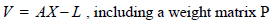Where:

V = matrix containing the image coordinate residuals

A = matrix containing the partial derivatives with respect to the unknown parameters, including exterior orientation, interior orientation, XYZ tie point, and GCP coordinates

X = matrix containing the corrections to the unknown parameters

L = matrix containing the input observations (that is, image coordinates and GCP coordinates)

The components of the least squares condition are directly related to the functional model based on collinearity equations. The A matrix is formed by differentiating the functional model, which is based on collinearity equations, with respect to the unknown parameters such as exterior orientation, and so forth. The L matrix is formed by subtracting the initial results obtained from the functional model with newly estimated results determined from a new iteration of processing. The X matrix contains the corrections to the unknown exterior orientation parameters. The X matrix is calculated in the following manner: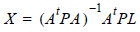Where:

X = matrix containing the corrections to the unknown parameters t

A = matrix containing the partial derivatives with respect to the unknown parameters

t = matrix transposed

P = matrix containing the weights of the observations

L = matrix containing the observations

Once a least squares iteration of processing is completed, the corrections to the unknown parameters are added to the initial estimates. For example, if initial approximations to exterior orientation are provided from Airborne GPS and INS information, the estimated corrections computed from the least squares adjustment are added to the initial value to compute the updated exterior orientation values. This iterative process of least squares adjustment continues until the corrections to the unknown parameters are less than a user-specified threshold (commonly referred to as a convergence value).

The V residual matrix is computed at the end of each iteration of processing. Once an iteration is completed, the new estimates for the unknown parameters are used to recompute the input observations such as the image coordinate values. The difference between the initial measurements and the new estimates is obtained to provide the residuals. Residuals provide preliminary indications of the accuracy of a solution. The residual values indicate the degree to which a particular observation (input) fits with the functional model. For example, the image residuals have the capability of reflecting GCP collection in the field. After each successive iteration of processing, the residuals become smaller until they are satisfactorily minimized.

Once the least squares adjustment is completed, the block triangulation results include:

• Final exterior orientation parameters of each image in a block and their accuracy
• Final interior orientation parameters of each image in a block and their accuracy
• X, Y, and Z tie point coordinates and their accuracy
• Adjusted GCP coordinates and their residuals
• Image coordinate residuals

The results from the block triangulation are then used as the primary input for the following tasks:

• Stereo pair creation
• Feature collection
• Highly accurate point determination
• DEM extraction
• Orthorectification

Normally, there are more or less systematic errors related to the imaging and processing system, such as lens distortion, film distortion, atmosphere refraction, scanner errors, and so forth. These errors reduce the accuracy of triangulation results, especially in dealing with large-scale imagery and high accuracy triangulation. There are several ways to reduce the influences of the systematic errors, like a posteriori-compensation, test-field calibration, and the most common approach: self-calibration (Konecny and Lehmann, 1984; Wang, Z., 1990).

The self-calibrating methods use additional parameters in the triangulation process to eliminate the systematic errors. How well it works depends on many factors such as the strength of the block (overlap amount, crossing flight lines), the GCP and tie point distribution and amount, the size of systematic errors versus random errors, the significance of the additional parameters, the correlation between additional parameters, and other unknowns.

There was intensive research and development for additional parameter models in photogrammetry in the 1970s -1980s, and many research results are available (for example, Bauer and Müller, 1972; Brown 1975; Ebner, 1976; Grün, 1978; Jacobsen, 1980; Jacobsen, 1982; Li, 1985; Wang, Y., 1988a, Stojic et al, 1998). Based on these scientific reports, IMAGINE Photogrammetry Project Manager provides four groups of additional parameters for you to choose for different triangulation circumstances. In addition, IMAGINE Photogrammetry Project Manager allows the interior orientation parameters to be analytically calibrated within its self-calibrating bundle block adjustment capability.

Automatic Gross Error Detection

Normal random errors are subject to statistical normal distribution. In contrast, gross errors refer to errors that are large and are not subject to normal distribution. The gross errors among the input data for triangulation can lead to unreliable results. Research during the 1980s in photogrammetric community resulted in significant achievements in automatic gross error detection in the triangulation process (for example, Kubik, 1982; Li, 1983; Li, 1985; Jacobsen, 1984; El-Hakim and Ziemann, 1984; Wang, Y., 1988a).

Methods for gross error detection began with residual checking using data-snooping and were later extended to robust estimation (Wang, Z., 1990). The most common robust estimation method is the iteration with selective weight functions. Based on the scientific research results from the photogrammetric community, IMAGINE Photogrammetry Project Manager offers two robust error detection methods within the triangulation process.

It is worth mentioning that the effect of the automatic error detection depends not only on the mathematical model, but also depends on the redundancy in the block. Therefore, more tie points in more overlap areas contribute better gross error detection. In addition, inaccurate GCPs can distribute their errors to correct tie points, therefore the ground and image coordinates of GCPs should have better accuracy than tie points when comparing them within the same scale space.